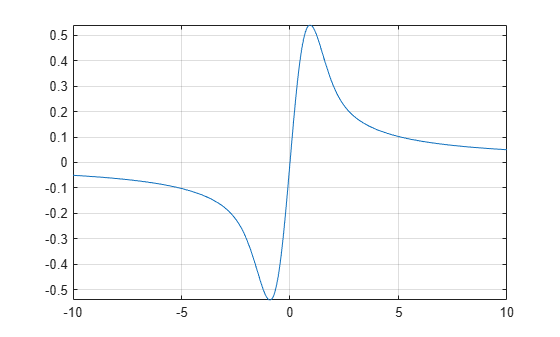# dawson

Dawson integral

## Syntax

``dawson(X)``

## Description

example

````dawson(X)` represents the Dawson integral.```

## Examples

### Dawson Integral for Numeric and Symbolic Arguments

Depending on its arguments, `dawson` returns floating-point or exact symbolic results.

Compute the Dawson integrals for these numbers. Because these numbers are not symbolic objects, `dawson` returns floating-point results.

`A = dawson([-Inf, -3/2, -1, 0, 2, Inf])`
```A = 0 -0.4282 -0.5381 0 0.3013 0```

Compute the Dawson integrals for the numbers converted to symbolic objects. For many symbolic (exact) numbers, `dawson` returns unresolved symbolic calls.

`symA = dawson(sym([-Inf, -3/2, -1, 0, 2, Inf]))`
```symA = [ 0, -dawson(3/2), -dawson(1), 0, dawson(2), 0]```

Use `vpa` to approximate symbolic results with floating-point numbers:

`vpa(symA)`
```ans = [ 0,... -0.42824907108539862547719010515175,... -0.53807950691276841913638742040756,... 0,... 0.30134038892379196603466443928642,... 0]```

### Plot the Dawson Integral

Plot the Dawson integral on the interval from -10 to 10.

```syms x fplot(dawson(x),[-10 10]) grid on```### Handle Expressions Containing Dawson Integral

Many functions, such as `diff` and `limit`, can handle expressions containing `dawson`.

Find the first and second derivatives of the Dawson integral:

```syms x diff(dawson(x), x) diff(dawson(x), x, x)```
```ans = 1 - 2*x*dawson(x) ans = 2*x*(2*x*dawson(x) - 1) - 2*dawson(x)```

Find the limit of this expression involving `dawson`:

`limit(x*dawson(x), Inf)`
```ans = 1/2```

## Input Arguments

collapse all

Input, specified as a symbolic number, variable, expression, or function, or as a vector or matrix of symbolic numbers, variables, expressions, or functions.

collapse all

### Dawson Integral

The Dawson integral, also called the Dawson function, is defined as follows:

`$\text{dawson}\left(x\right)=D\left(x\right)={e}^{-{x}^{2}}\underset{0}{\overset{x}{\int }}{e}^{{t}^{2}}dt$`

Symbolic Math Toolbox™ uses this definition to implement `dawson`.

The alternative definition of the Dawson integral is

`$D\left(x\right)={e}^{{x}^{2}}\underset{0}{\overset{x}{\int }}{e}^{-{t}^{2}}dt$`

## Tips

• `dawson(0)` returns `0`.

• `dawson(Inf)` returns `0`.

• `dawson(-Inf)` returns `0`.

## Version History

Introduced in R2014a